# 介绍# 2.系统总览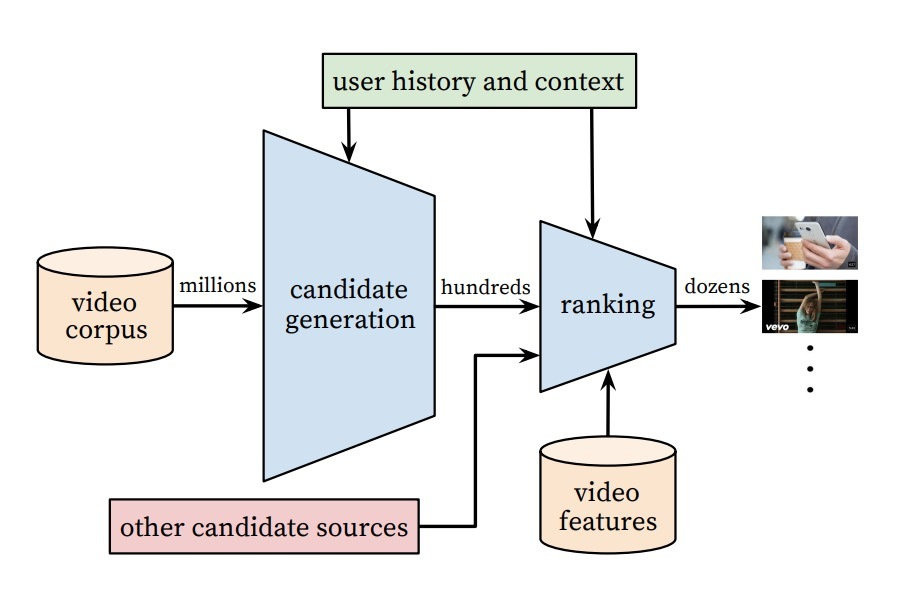# 一、候选生成

## 3.1 推荐即分类

Efficient Extreme Multiclass

## 1.1 模型架构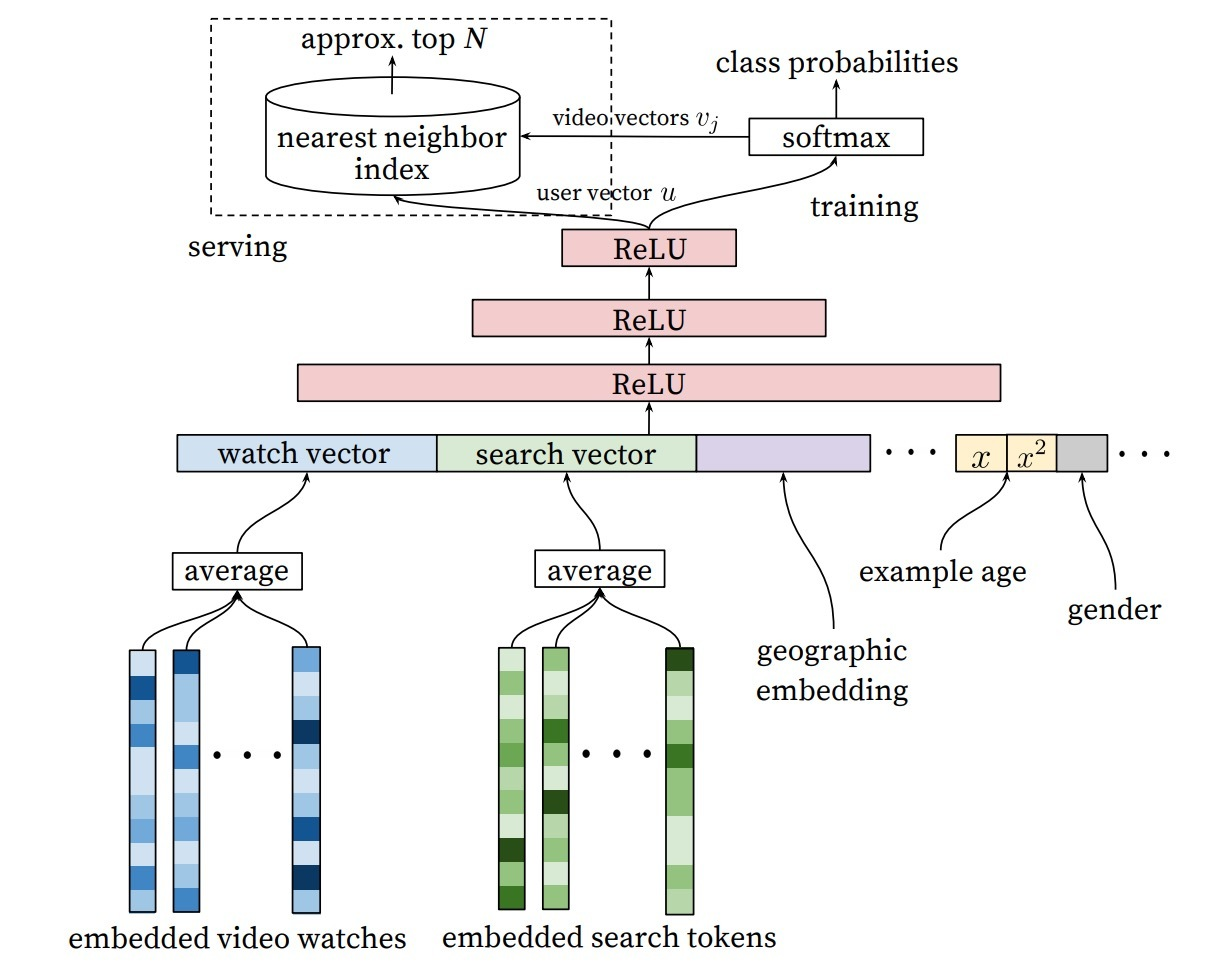## 1.2 多种信号

“样本时限”特征（”Example Age” Feature）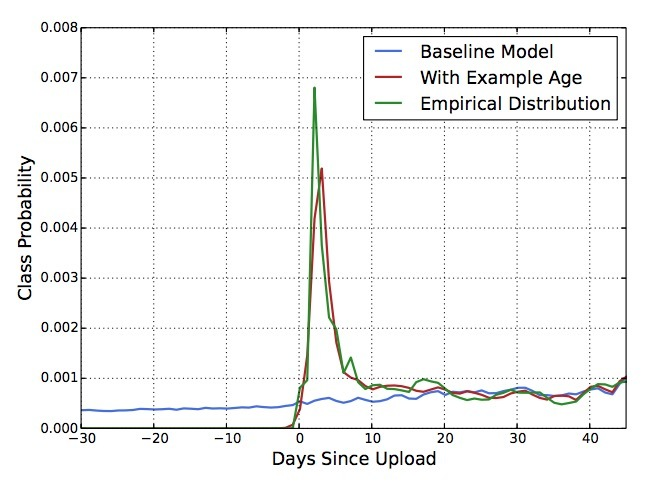## 1.3 Label和Context选择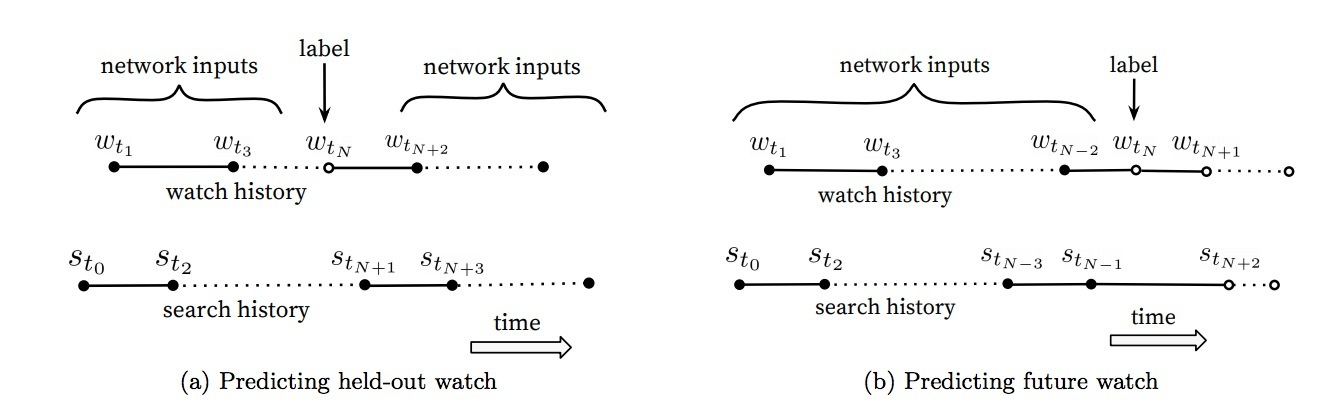## 1.4 特征和深度的试验

• Depth 0: 一个线性层，可简单地将串联层转换成与softmax相匹配的256维.
• Depth 1: 256 ReLU
• Depth 2: 512 ReLU -> 256 ReLU
• Depth 3: 1024 ReLU -> 512 ReLU -> 256 ReLU
• Depth 4: 2048 ReLU -> 1024 ReLU -> 512 ReLU -> 256 ReLU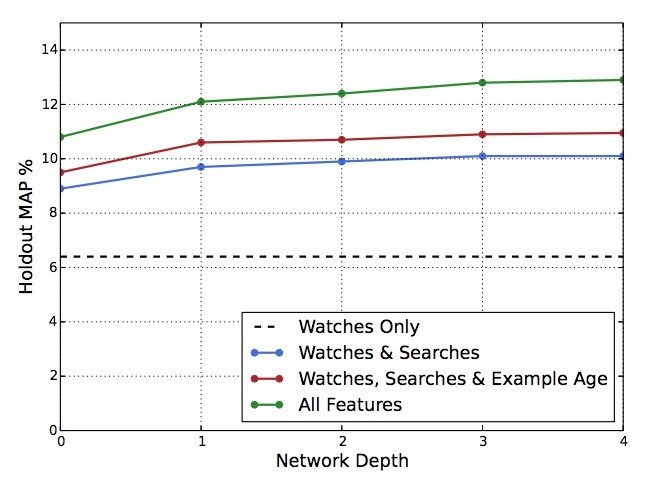# 二、Ranking

Ranking的主要作用是，针对指定的UI，使用曝光数据来特化和校正候选预测（specialized and calibrate candidate predictions）。例如，用户通常会观看一个probability值较高的视频，但不大可能去点击指定主页上缩略图的曝光。在Ranking时，我们会访问许多描述视频的特征、以及视频与用户关系的特征，因为在候选集生成阶段，只有一小部分的视频被打过分，而非上百w的视频。Ranking对于聚合不同的候选源很重要，因为每个源的得分不能直接对比。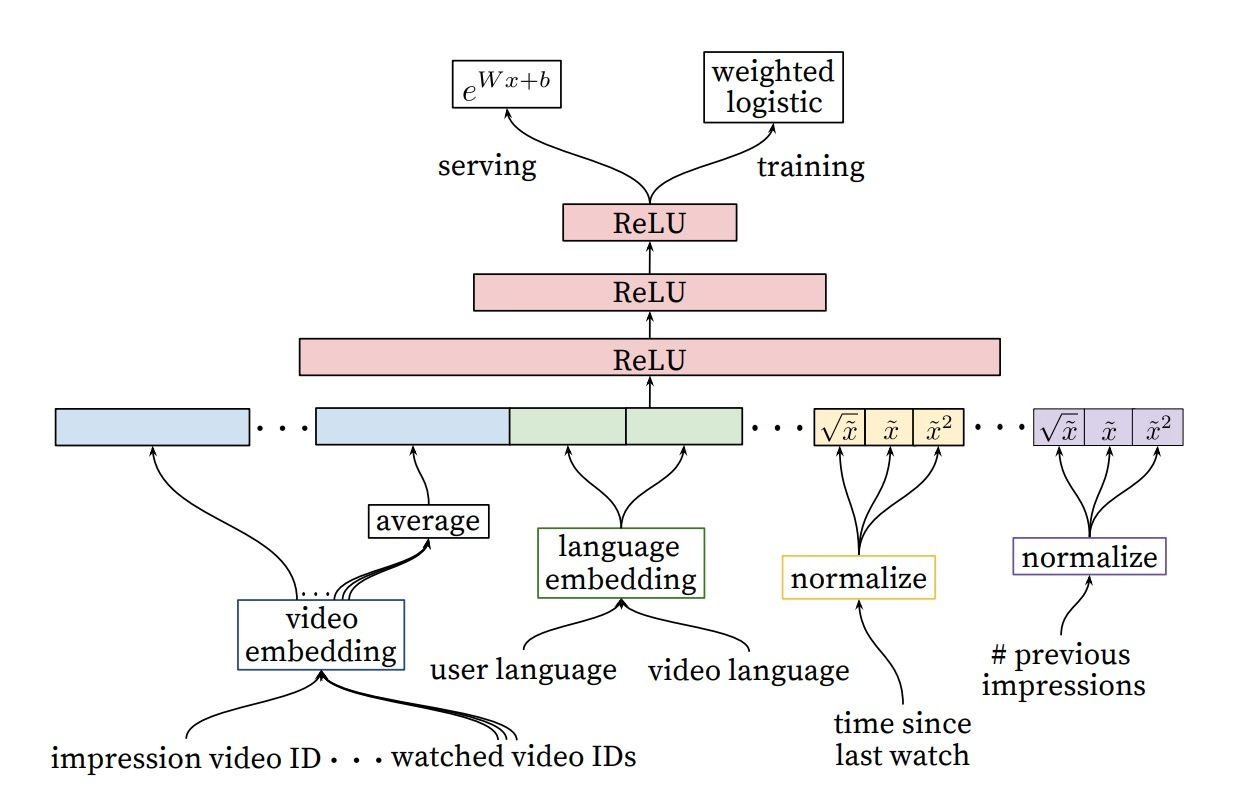## 2.3 隐层的试验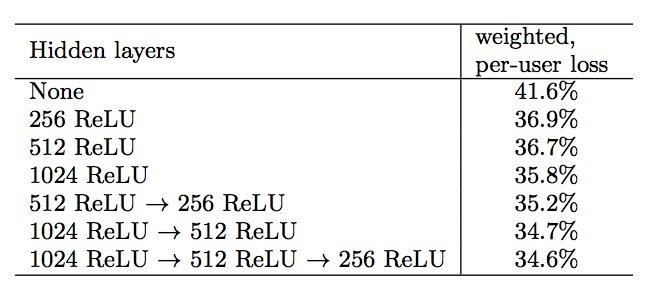# 3.层次化语言模型

## 3.1 模型结构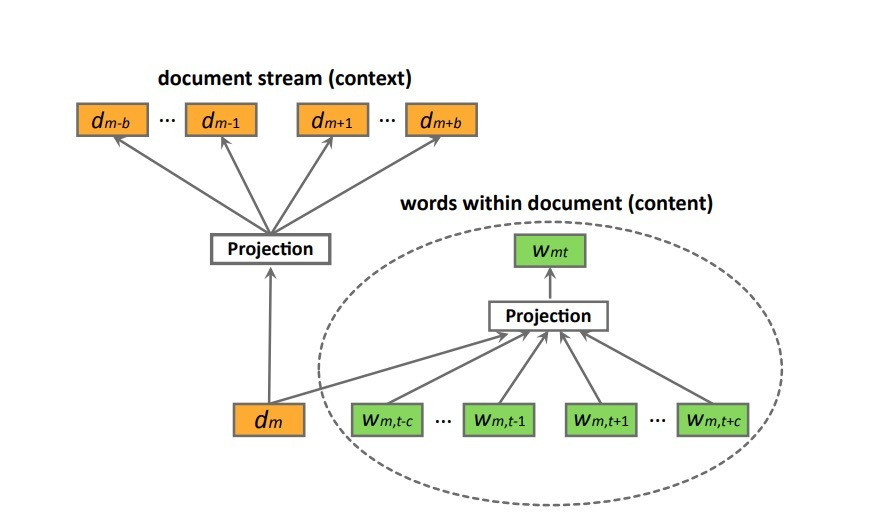$L = \sum_{s \in S} (\alpha \sum_{d_m \in s} \sum_{w_{mt} \in d_m} log P(w_{mt} | w_{m,t-c}: w_{m,t+c}, d) + \\ \sum _{d_m \in s} (\alpha log P(d_m | w_{m1}: w_{mT}) + \sum_{-b \le i \le b, i \neq 0} log P(d_{m+i} | d_m)))$ …(8)

$P(d_{m+i} | d_{m}) = \frac{exp(v_{d_m}^T v_{d_{m+i}}')} {\sum_{d=1}^{M} exp(v_{d_m}^T v_d')}$ …(9)

$P(w_{mt} | w_{m,t-c}: w_{m,t+c}, d_m) = \frac{exp(\bar{v}^T v_{w_{mt}}')} {\sum_{w=1}^{W} exp(\bar{v}^T v_w')}$ …(10)

$\bar{v} = \frac{1}{2c+1} (v_{d_m} + \sum_{-c \leq j \leq c, j \neq 0} v_{w_{m,t+j}})$ …(11)

## 3.3 模型最优化

$P(d_{m+i} \mid d_m) = \prod_l P(h_l \mid q_l, d_m)$ …(12)

$P(h_l = 1 \mid q_l, d_m) = \sigma(v_{d_m}^T V_{q_l})$ …(13)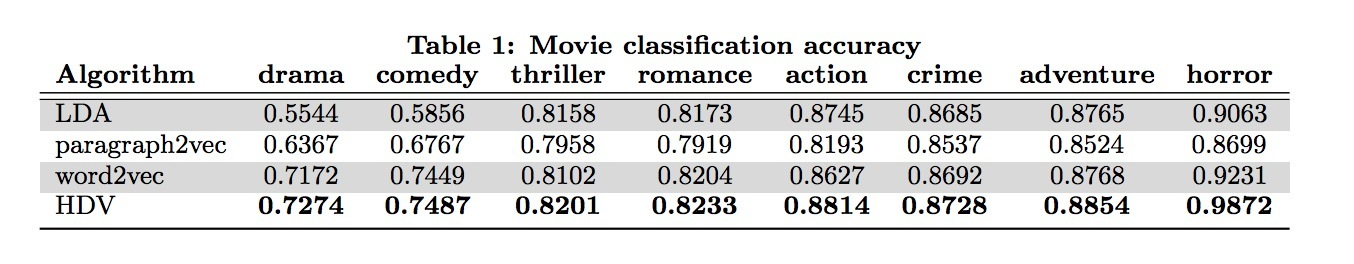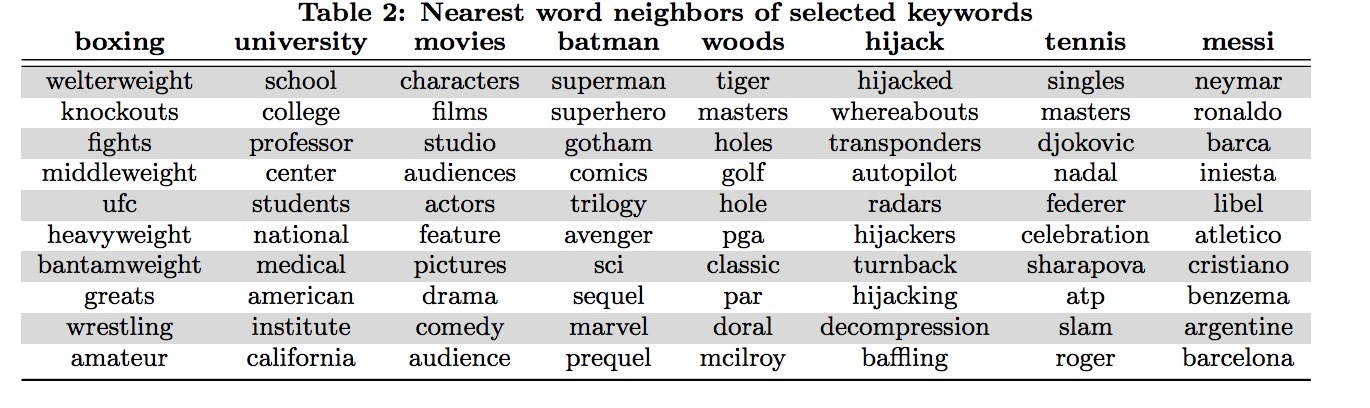# 1.介绍DeepWalk会将一个图作为输入，并生成一个隐表示作为它的输出。将我们的方法应用于Karate network上，结果如图1所示。图1a通过力导向（force-directed）的布局进行表示，图1b展示了我们使用2个隐维度的方法。除了显著的相似性外，我们注意到1b中线性可分的部分，对应于通过输入图1a的模块最大化（modularity maximization）的聚类（clusters）: 通过顶点颜色区分。

DeepWalk比其它用于创建社交维度（social dimension）隐表示方法要好，尤其是当带label的节点（labeled nodes）很稀少时。我们的表示法的性能可能与最简单的分类器（LR）相当。我们的表示是可泛化的，可以结合任何分类方法（包含迭代型推断法）。DeepWalk可以完成上述所有，它是一个在线算法，可并行化。

• 引入深度学习作为一个工具来分析图，来构建健壮的表示，很适合于统计建模。DeepWalk会学到在短随机游走(short random walks)内表示的结构化规律。
• 我们对多个社交网络上使用我们的表示法，并在多标签分类上进行评估。我们发现可以极大提升存在标签稀疏性情况的分类效果，在Micro F1上可以提升5%-10%。在一些case中，即使只给了更少的60%的数据，DeepWalk的表示也可以胜出其它对手。

# 3.学习社交表示

• 社群意识（Community aware）：隐维度间的距离可以作为评估网络成员的社交相似性的一个metric。这允许泛化到同质的网络中。
• 低维（Low dimensional）：当标记数据很少时，低维模型泛化更好，加速收敛和推断。
• 连续（Continuous）：我们需要隐表示来建模在连续空间中的部分社群成员关系（community membership）。除了提供一个关于社群关系的细微视图外，连续表示法具有在社群间的平滑决策边界，这可以允许更健壮的分类。

## 3.1 随机游走

• 1.局部探索（local exploration）很容易并行化。多个随机游走者（random walkers）以不同线程、进程、机器可以并行探索同一个graph下的不同部分。
• 2.依靠从短随机游走上获取信息，可以使它更能适应在图结构上的小变动，无需进行全局重新计算。我们可以迭代式地更新学到的模型，从变动区域上使用新的随机游走，它对整个graph是在时间上是次线性的。

## 3.2 连接：二八法则（power law）## 3.3 语言建模

$Pr(v_i | (\Phi(v_1), \Phi(v_2), ..., \Phi(v_{i-1})))$ …(1)

$minimize_{\Phi} - log Pr({v_{i-w}, ..., v_{i-1}, v_{i+1}, ..., v_{i+w}} | \Phi(v_i))$ …(2)

# 4.方法

## 4.2 算法：DeepWalk

• 1.随机游走生成器
• 2.一个更新过程### 4.2.1 SkipGram

SkipGram是一个语言模型，在一个句子中，它会最大化在同一窗口w中词之间的共现率。## 4.3 并行化(Parallelizability)# 参考

DeepWalk: Online Learning of Social Representations

# 1.介绍# 2.方法

## 2.1 引入skip-ghought vectors

Encoder：假设$w_i^1, ..., w_i^N$是句子$s_i$中的words，其中N表示在句子中的words数目。在每个step中，encoder会产生一个hidden state：$h_i^t$，它可以解释成序列$w_i^1,...,w_i^t$的表示（representation）。hidden state：$h_i^N$可以表示整个句子。

$r^t = \sigma(W_r x^t + U_r h^{t-1})$ … (1)

$z^t = \sigma(W_z x^t + U_z h^{t-1})$ … (2)

$\bar{h}^t = tanh(W x^t + U (r^t \odot h^{t-1})$ … (3)

$h^t = (1-z^t) \odot h^{t-1} + z^t \odot \bar{h}^t$ …(4)

Decoder: decoder是一个神经语言模型，它的条件是encoder output $h_i$。该计算与encoder相似，除了我们引入了矩阵$C_z，C_r$，以及C，它们被用于偏置由句子向量计算得到的update gate，reset gate和hidden state。一个decoder会被用于下一个句子$s_{i+1}$，而第二个decoder被用于前一句子$s_{i-1}$。Separate参数被用于每个decoder，除了词汇矩阵V，它的权重矩阵会连接decoder的hidden state，以便计算在词上的一个分布。我们在下一个句子$s_{i+1}$上描述decoder，通过一个在前一句子$s_{i-1}$上的类似计算得到。假设$h_{i+1}^t$表示decoder在时间t的hidden state。对下面的等式进行迭代（丢掉下标i+1）：

$r^t = \sigma(W_r^d x^{t-1} + U_r^d h^{t-1} + C_r h_i)$ …(5)

$z^t = \sigma(W_z^d x^{t-1} + U_z^d h^{t-1} + C_z h_i)$ …(6)

$\bar{h}^t = tanh(W^d x^{t-1} + U^d (r^t \odot h^{t-1} + C h_i$ …(7)

$h_{i+1}^t = (1-z^t) \odot h^{t-1} + z^t \odot \bar{h}^t$ …(8)

$% $ …(9)

$% $ …(10)

# 3 实验

• 使用学到的encoder作为一个feature extractor，抽取所有句子的skip-thought vectors
• 如果该任务涉及到计算句子对（pairs of sentences）之间的分数，会计算pairs间的component-wise features。
• 在抽取的features上训练一个线性分类器，在skip-thoughts模型中没有任何额外的fine-tuning或者backpropagation。

t-sne.

# 参考

Convolutional Neural Networks for Sentence Classification

# rank fusion介绍

《Web Metasearch: Rank vs. Score Based Rank Aggregation Methods》提出了rank fusion的问题。

# 1.介绍

rank fusion的问题是，给定多个judges的独立ranking偏好（一个ranking是一个items集合的线性顺序），计算一个“一致的（consensus）”ranking。该ranking fusion问题出现在许多情况下，一个著名的场景是metasearch：metasearch会处理由多个search engines对于一个给定query所返回的多个result lists组合，其中，在result list中的每个item对于各自的search engine是有序的，并且有一个相关分值。

• ii) 有许多信息源
• iii) 对于一些搜索引擎，广告商付费越多，在search results上的rank会越高，这被称为：“pay-for-placement”，这在precesion上会有平均损失
• iv) search engines会有作弊（spamming）

ranking fusion的理想场景是，每个judge（search engine）给出对所有alternative items一个完整顺序。不幸的是，在metasearch中这不太现实，有两个原因：

• i) search engines的coverage是不同的
• ii) search engines会限制访问top 100 or 1000个ranked items

。。。

# 2.ranking fusion方法

• i) 顺序排序(irdinal rank)：rank list中的一个item分配一个顺序
• ii) 得分(score)：在rank list中分配给每个item的得分

## 2.1 前提

• U是一个items集合，称为“universe”。
• $\tau$是一个rank list，它与U有关，是关于子集$S \subseteq U$的一个排序，例如：$\tau = [x_1 \geq x_2 \geq \cdots \geq x_k$，对于每个$x_i \in S$$\geq$是在S上的顺序关系.

。。。

## 2.2 Fusion方法

• U表示在$\tau_1, \cdots, \tau_n$中的items的union，例如：$U = \cup_{\tau \in R, i \in \tau} \lbrace i \rbrace$
• $\hat{\tau}$表示ranking（被称为fused ranking或fused rank list），它是一个rank fusion方法应用到在R中的rank lists之后得到的结果。

• 如果两个fused ranking $\hat{\tau}_1, \hat{\tau}_2$相等的（equal），则：$\hat{\tau}_1 = \hat{\tau}_2$
• 如果$\hat{\tau}_1$$\hat{\tau}_2$等价的(equivalent)，那么对于$i \in U$，有$\hat{\tau}_1(i) = \hat{\tau}_2(i)$（他们具有相同的顺序）

### 2.2.1 线性组合

Fox提出的ranking fusion方法基于对每个item的归一化得分(normalised score)采用不加权（unweighted）的min, max或sum。另外，Lee解决了rank取代score的case。两种方法如下：

。。。

#### 2.2.1.1 Borda Count

Borda的方法[2,14]是一个基于ranks的voting方法，例如，一个candidate出现在每个voter的ranked list中，则会为相应的ranks分配一个weight。计算上非常简单，因为实现是线性的。Borda Count (BC)方法在rank fusion问题中被引入，并以如下方式运转。每个voter会对一个关于c个candidates的固定集合以偏好顺序进行rank。对于每个voter，会给top ranked candidate为c分，第二个ranked candidate会得到c-1分，以此类推。在unranked candidates间则均匀分配剩余得分。candidates会以总分(total points)的递减顺序进行rank。正式的，该方法与以下过程等价：对于每个item $i \in U$以及rank list $\tau \in R$，Borda归一化权重为$w^{\tau}(i)$（定义1）。fused rank list $\hat{\tau}$根据Borda score $s^{\hat{\tau}}$的顺序排序，其中在$\hat{\tau}$中的一个item $i \in U$被定义为：

…(6)

### 2.2.2 Markovchain-based方法

中提出一种关于rank fusion的有意思的方法，它基于Markov chains实现。一个系统的一个(齐次：homogeneous)Markov chain可以通过一个状态集$S=\lbrace 1,2, \cdots, \mid S \mid \rbrace$以及一个$\mid S \mid \times \mid S \mid$的非负随机矩阵M（例如：每行的求和为1）来指定。该系统从S中的某个状态开始，并在每个step时会从一个state转移到另一个state。转移(transition)通过矩阵M来指导：在每个step上，如果系统从状态i移到状态j的概率为$M_{ij}$。如果给定当前状态作为概率分布，下一状态的概率分布通过表示当前状态的该vector与M相乘得到。总之，系统的起始状态（start state）根据在S上的一些分布x被选中。在m个steps后，该系统的状态会根据$xM^m$进行分布。在一些条件下，不需要考虑起始分布x，该系统最终会达到一个唯一确定点（状态分布不再变化）。该分布称为“稳态分布(stationary distribution)”。该分布可以通过M的左主特征向量（principal left eigenvector）y给出，例如：$yM = \lambda y$。实际上，一个简单的power-iteration算法可以快速获得关于y的一个合理近似。y中的entries定义了在S上的一个天然顺序。我们称这样的顺序为M的马尔可夫排序（Markov chain ordering）

• $MC_1$: 如果当前state为item i，那么，下一state从对应rank >= item i的所有items j的multiset中均匀选中，例如，从multiset $Q_i^{C_1} = \cup_{k=1}^n \lbrace j: \tau_k(j) \leq \tau_k(i) \rbrace$中均匀选中下一state。

• $MC_2$：如果当前state为item i，下一state的挑选过程如下：首先从所有包含i的$\tau_1, \cdots, \tau_n$中均匀选中一个ranking $\tau$，接着从集合$Q_{\tau,i}^{C_2} = \lbrace \tau(j) \leq \tau(i) \rbrace$中均匀选中一个item j

• $MC_3$：如果当前state为item i，下一state的挑选过程如下：首先从包含i的所有$\tau_1, \cdots, \tau_n$中均匀选择一个ranking $\tau$，接着均匀选择通过$\tau$排序的一个item j。如果$% $那么跳到j，否则留在i；

• $MC_4$：如果当前state为item i，下一state挑选过程如下：首先从S中均匀选中一个item j。如果$% $对于会同时对i和j进行排序的lists $\tau \in R$中的大多数都成立，那么跳到j，否则留在i。• $M_{13}^1$是2/6. 确实，$Q_1^{C_1}$$\lbrace 1,1,3,1,2,3 \rbrace$(rank<=item 1的case：1; 1,3; 1,2,3)。因此，在$Q_1^{C_1}$中均匀选中某一元素的概率为1/6, 选中item 3的概率为2/6.

• $M_{21}^2$是5/18. 均匀选择一个包含item 2的rank list的概率为1/3. 如果$\tau_1$被选中，那么$Q_{\tau_1,2}^{C_2}=\lbrace 2,1 \rbrace$。因此，选中item 1的概率为1/2. 相似的，有$Q_{\tau_2,2}^{C_2}=\lbrace 2,1,3 \rbrace$，以及$Q_{\tau_3,2}^{C_2}=\lbrace 2, 3 \rbrace$。因此，$M_{21}^2 = \frac{1}{3} \cdot \frac{1}{2} + \frac{1}{3} \cdot \frac{1}{3} + \frac{1}{3} \cdot 0 = \frac{5}{18}$

• $M_{23}^3$是2/9. 均匀选中一个包含item 2的概率为1/3. 在一个rank list范围内均匀选择一个item的概率也为1/3. 由于$% $, 因此$M_{23}^3 = \frac{1}{3} \cdot 0 + \frac{1}{3} \cdot \frac{1}{3} + \frac{1}{3} \cdot \frac{1}{3} = \frac{2}{9}$• $M_{22}^4$是1/3. 均匀选中在S中一个item的概率为1/3. 另外，考虑图3中的表：在上表中的每个entry $a_{ij}$是满足$% $的lists的count数（例如，有多少rankings满足：item j的rank比item i要好）。由于存在三个lists，因此majority的阀值是2. $M_{22}^4$指的是：给定item 2, 在下一step之后我们仍停留在item 2上的概率。由于$a_{21}, a_{22}, a_{23}$分别是2､ 0､ 2, 三种情况中有两种会从item 2进行转移，另一种仍会停留在item 2上。相应的$M_{22}^4$是1/3.